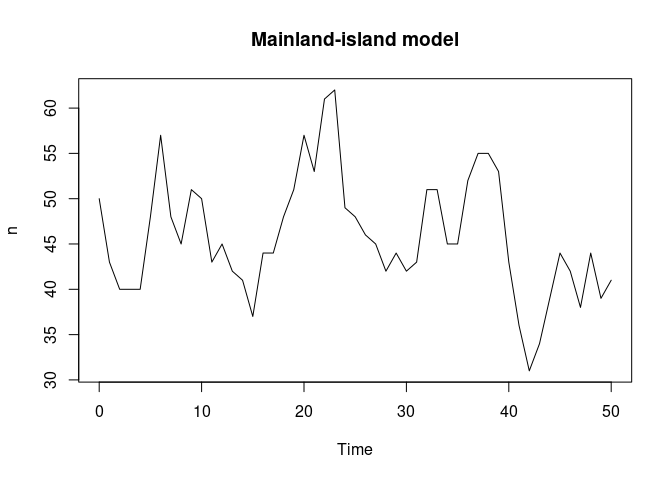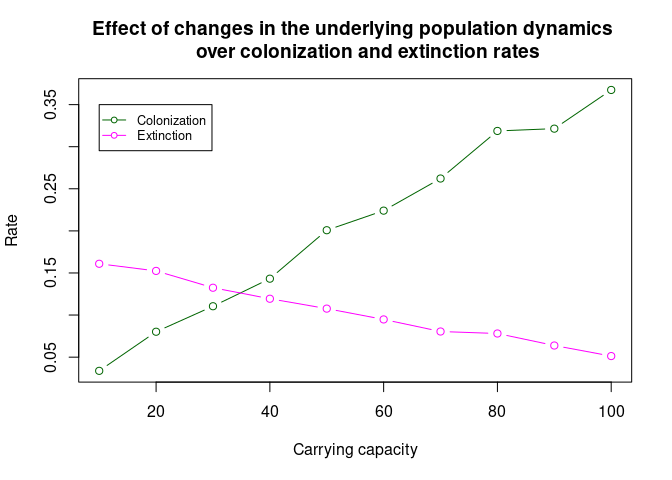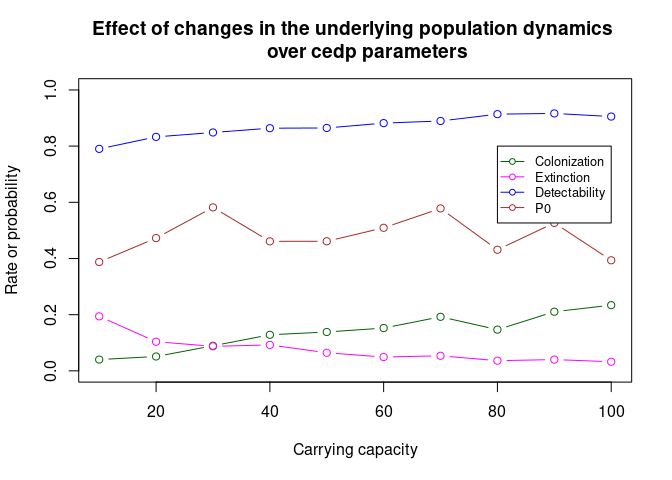# Colonization and extinction rates for IBD models

## Inmigration, Birth and Death stochastic models.

Colonization and extinction rates can be considered effective rates in the sense that they are average approximations to the intrinsic individual-level processes driving species-specific dynamics. This function includes three simple stochastic population models simulated with Gillepie’s exact algorithm (Gillespie 1977). All three models assume individuals as discrete entities subject to a range of processes. Our aim is to show the correspondence between parameters driving individual deaths and births, and colonization-extinction rates at the population level. The population models included in function ibd_models are: the seminal model by Kendall (1948), a mainland-island model by Alonso & McKane (2002) and a basic population model with density-dependent deaths by Haegeman & Loreau (2010). The three models have three basic processes that differ in their parametrization: inmigration (arrival of new individuals from outside the population), individual births and individual deaths. In the following table, we specify the processes and the transitions (with probability rates in units of Time⁻¹) associated with each model.

Process Transition Kendall (1948) Alonso & McKane (2002) Haegeman & Loreau (2010)
Inmigration $$\emptyset \rightarrow A$$ $$\mu$$ $$\mu (K - n)$$ $$\mu$$
Birth $$A \rightarrow A + A$$ $$\beta n$$ $$\beta n (1 - \frac{n}{K})$$ $$\beta n$$
Death $$A \rightarrow \emptyset$$ $$\delta n$$ $$\delta n$$ $$n (\delta + (\beta - \delta) \frac{n}{K})$$

Please note that Kendall’s model may explode if the birth rate is higher than the death rate. Notice also that the death rate in the Haegeman & Loreau model may become ill-defined, this is, negative for death rate values higher than the value for the birth rate, given that probability rates can never be negative.

## Simulation of stochastic population dynamics

The function ibd_models allows to simulate the stochastic population dynamics described in the previous section. The function requires that we specify arguments n0 (initial population size), beta (birth rate), delta (death rate), mu (inmigration rate), and K (carrying capacity) when required. We also need to specify a vector of sampling times time_v and the model we plan to use via argument type. To illustrate its stochastic nature, the following code simulates the dynamics of Alonso and McKane (2002) model, with equal birth and death rates and low inmigration.

set.seed(101100111)
dynamic <- ibd_models(n0 = 50, beta = 0.3, delta = 0.3, mu = 0.01, K = 300, time_v = 0:50, type = "Alonso")
plot(dynamic, type = "l", main = "Mainland-island model")## Colonization and extinction rates derived from the individual-based, stochastic dynamics of the population

In the following section, we illustrate the use of colonization and extinction rates as effective parameters reflecting the underlying species dynamics. We used the Alonso & McKane model implemented in function ibd_models to examine the effect of varying carrying capacity $$K$$ on our colonization and extinction rates for a given set of parameters, showing that changes in carrying capacity can drastically change the colonization and extinction rates obtained.

ts <- 0:100 #Time-vector
ccc <- seq(10, 100, 10) #Carrying capacities
out <- NULL #Initializing output
for (i in ccc){
pops <- matrix(nrow = 100, ncol = 101)
for (j in 1:100){
sim <- ibd_models(n0 = 0, beta = 0.2, delta = 0.3, mu = 0.004, K = i, time_v = ts,
type = "Alonso") #Simulations
pops[j, ] <- (sim[, 2] > 0) * 1.0
}
out <- rbind(out, c(i, regular_sampling_scheme(pops, 1:101)))
}

#Plotting
plot(out[, 1], out[, 2], type = "b", xlab = "Carrying capacity", ylab = "Rate",
col = "darkgreen", main = "Effect of changes in the underlying population dynamics
over colonization and extinction rates")
lines(out[, 1], out[, 5], type = "b", col = "magenta")
legend(10, .35, legend=c("Colonization", "Extinction"),
col=c("darkgreen", "magenta"), pch = 21, lty=1, pt.bg = "White",  cex=0.8)In order to generate more realistic simulations, we can add a detectability filter to the output of function ibd_models. For the sake of simplicity, we assume that the detectability of a species is a function of the number of individuals present in the population, as described by the following equation: $d_n = 1 - (1 - d) ^ n$ where $$d_n$$ is the probability of finding the species, $$d$$ is the detection probability of one individual, and $$n$$ is the number of individuals present in the population. This assumes that individuals are detected independently from each other with the same constant probability $$d$$.
The next example follows the same procedure as in the previous section, with the added step of sampling population at some detectability level. It illustrates how changes in population-level parameters make an influence on colonization and extinction estimates.

ts <-  seq(0, 49, 7) #Time-vector
ccc <- seq(10, 100, 10) #Carrying capacities
out <- NULL
for (i in ccc){
pops <- matrix(nrow = 100, ncol = 24)
for (j in 1:100){
sim <- ibd_models(n0 = sample(c(0,1), 1), beta = 0.24, delta = 0.3, mu = 0.004, K = i, time_v = ts,
type = "Alonso")

# Applying the detectability filter and preparing the data for sss_cedp
filter1 <- (runif(8) < 1 - (1 - 0.7)^sim[, 2]) * 1.0
filter2 <- (runif(8) < 1 - (1 - 0.7)^sim[, 2]) * 1.0
filter3 <- (runif(8) < 1 - (1 - 0.7)^sim[, 2]) * 1.0
filtered <- cbind(filter1, filter2, filter3)
filtered2 <- c(t(filtered))
pops[j, ] <- filtered2
}

out <- rbind(out, c(i, unlist(sss_cedp(pops, seq(0, 49, 7), rep(3, 8), Colonization = 0.1, Extinction = 0.1, Phi_Time_0 = 0.5, Detectability = 0.7))))
}

plot(out[, 1], out[, 2], type = "b", xlab = "Carrying capacity", ylab = "Rate or probability",
col = "darkgreen", main = "Effect of changes in the underlying population dynamics
over cedp parameters", ylim = c(0, 1))
lines(out[, 1], out[, 3], type = "b", col = "magenta")
lines(out[, 1], out[, 4], type = "b", col = "blue")
lines(out[, 1], out[, 5], type = "b", col = "brown")
legend(80, .8, legend=c("Colonization", "Extinction", "Detectability", "P0"),
col=c("darkgreen", "magenta", "blue", "brown"), pch = 21, lty=1, pt.bg = "White",  cex=0.8)